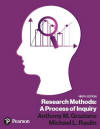﻿ Repeated Measures ANOVA in SPSS for WindowsGraziano & Raulin
Research Methods (9th edition)

## Repeated Measures ANOVA

The most commonly used statistical analysis for the single-variable, within-subjects experiment is a repeated measures ANOVA, which was introduced in Chapter 11. Unlike the one-way ANOVA, the repeated measures ANOVA is modified to take into account the fact that the conditions are not independent of each other but are correlated.

The advantage of a within-subjects design is that it effectively equates participants in different conditions prior to experimental manipulation by using the same participants in each condition. Therefore, the single largest contributing factor to error variance--individual differences--has been removed.

In the language of analysis of variance, we have removed the effects of individual differences from the error component. What effect do you think this would have on the F-ratio? Because the individual difference portion of the error term has been removed, the denominator in the F-ratio will be smaller and, therefore, the F will be larger. This means that the procedure will be more sensitive to small differences between groups.

In a repeated measures ANOVA, the total sum of squares is computed in the same way as in a simple, one-way ANOVA. What is called a between-groups sum of squares in a simple one-way ANOVA is, in this case, called a between-conditions sum of squares, or simply, a between sum of squares. Terminology is changed in the repeated measures design because there is only one group of subjects.

The within-groups sum of squares in the repeated measures ANOVA is split into two terms: subjects and error. The subjects term is the individual differences component of the within-groups variability. The error term is what is left of the within-groups variability after the individual differences component is removed.

In the repeated measures ANOVA, we test the null hypothesis that there are no differences between conditions by dividing the mean square between by the error mean square. As in the independent groups ANOVA, the ratio of mean squares is an F-ratio. The computational procedures for a repeated measures ANOVA are included on this website, although we recommend using a computer program to do the computations.

A repeated measures ANOVA is the appropriate analysis for the kind of data shown in Table 11.1 of the text. You can open this data file using the File menu and Open submenu. [The data file is on the website and can be downloaded to your computer.] This screen show how the data file looks.

Unfortunately, a repeated measures ANOVA is one of the few statistical procedures that is not included in the SPSS for Windows Student Version. It can be run with a more comprehensive version of SPSS for Windows, which may be available on public computers at your university. It can also be run by a variety of other statistical packages. We will briefly outline how this analysis is run from the more comprehensive version of SPSS for Windows.

We used a more comprehensive version of SPSS for Windows to compute the repeated measures ANOVA for the data listed in Table 11.1. This screen shows the data file for this example. Note that, unlike the data file for a one-way ANOVA in a between-subjects design, in which only one dependent measure appears on each line, in the repeated measures ANOVA each line contains a participant's scores on the dependent measure for each condition of the experiment. This difference in how the data file is set up can be confusing. However, there is an easy way to remember how to set up the file. Just remember that SPSS for Windows, and virtually every other statistical analysis package, will assume that each line (or record) represents the data from one participant. In a between-subjects design, each participant contributes one score; therefore, there is only one score per line. In a within-subjects design, each participant contributes scores in each condition; therefore, each line has the participant's score for each condition.

To compute the repeated measures ANOVA in the more comprehensive version of SPSS for Windows, we selected the Analyze menu, the ANOVA Models submenu, and the Repeated Measures option. The F-ratio in this example was 32.25 with a p-value of <.001. As with all statistical analyses, if the p-value is smaller than the alpha level we set (traditionally .05), we reject the null hypothesis.

The analysis of variance tests the null hypothesis that there are no differences between any of the conditions. Therefore, a significant F-ratio indicates that at least one of the condition means is significantly different from at least one other condition mean. To determine which means are significantly different from which other means, we must use one of the statistical tests to probe for specific mean differences. Computational procedures for the tests can be found in most advanced statistics textbooks (Keppel, 2006; Myers & Well, 2003). Most computerized statistical analysis packages include these tests as an option in the repeated measures analysis.